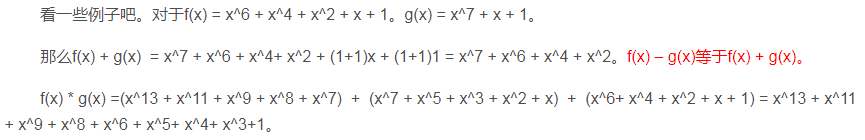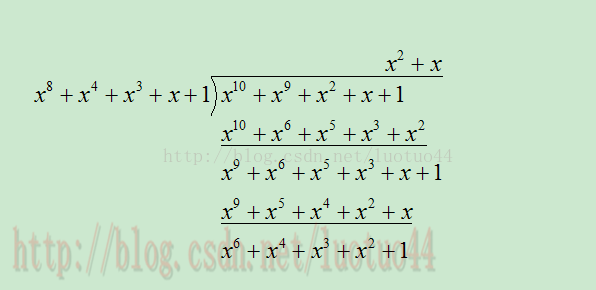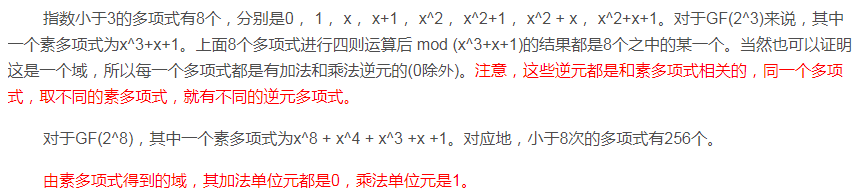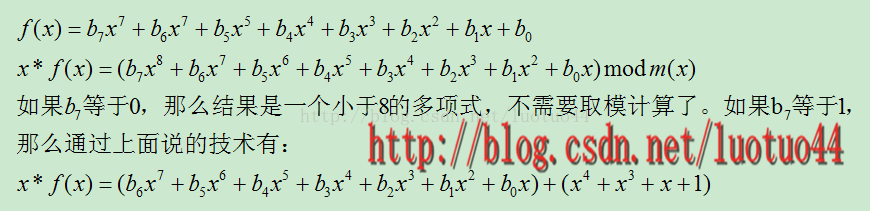# 1、有限域GF(2^8)的四则运算

//g_prime就是 GF(p)中的p
int g_prime = 251;

int calculateInverse(int x)
{
int a1 = 1, a2 = 0, a3 = g_prime;
int b1 = 0, b2 = 1, b3 = x;

while( 1 )
{
if( b3 == 0 )
throw std::logic_error("should not be 0");

if( b3 == 1 )
break;

int q = a3 / b3;

int t1 = a1 - q*b1, t2 = a2 - q*b2, t3 = a3 - q*b3;

a1 = b1; a2 = b2; a3 = b3;
b1 = t1; b2 = t2; b3 = t3;
}

return (b2 + g_prime)%g_prime;
}


现在重点讲一下GF(2^n)，特别是GF(2^8)，因为8刚好是一个字节的比特数。**素多项式模运算：GF(2^8)的四则运算：

    首先有，x^8 mod m(x) = [m(x) – x^8] = x^4 + x^3 +x +1。GF(2^n)是一个有限域，就是元素个数是有限的，但指数k是可以无穷的。所以必然存在循环。这个循环的周期是2^n-1，因为多项式0，g不能生成，少了一个。所以对于GF（2^8），当k大于等于255时，g^k =g^(k%255)。所以对于正表，生成元的指数，取0到254即可，对应地生成255个不同的多项式，多项式的取值范围为1到255。

int table;
int i;

table = 1;//g^0
for(i = 1; i < 255; ++i)//生成元为x + 1
{
//下面是m_table[i] = m_table[i-1] * (x + 1)的简写形式
table[i] = (table[i-1] << 1 ) ^ table[i-1];

//最高指数已经到了8，需要模上m(x)
if( table[i] & 0x100 )
{
table[i] ^= 0x11B;//用到了前面说到的乘法技巧
}
}


int arc_table;

for(i = 0; i < 255; ++i)
arc_table[ table[i] ] = i;


int inverse_table;

for(i = 1; i < 256; ++i)//0没有逆元，所以从1开始
{
int k = arc_table[i];
k = 255 - k;
k %= 255;//m_table的取值范围为 [0, 254]
inverse_table[i] = table[k];
}


int mul(int x, int y)
{
if( !x || !y )
return 0;

return table[ (arc_table[x] + arc_table[y]) % 255];
}


©️2019 CSDN 皮肤主题: 大白 设计师: CSDN官方博客